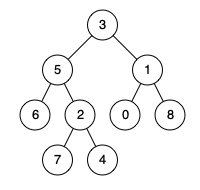##### Welcome to Subscribe On Youtube

Formatted question description: https://leetcode.ca/all/1740.html

# 1740. Find Distance in a Binary Tree

Medium

## Description

Given the root of a binary tree and two integers p and q, return the distance between the nodes of value p and value q in the tree.

The distance between two nodes is the number of edges on the path from one to the other.

Example 1:Input: root = [3,5,1,6,2,0,8,null,null,7,4], p = 5, q = 0

Output: 3

Explanation: There are 3 edges between 5 and 0: 5-3-1-0.

Example 2:Input: root = [3,5,1,6,2,0,8,null,null,7,4], p = 5, q = 7

Output: 2

Explanation: There are 2 edges between 5 and 7: 5-2-7.

Example 3:Input: root = [3,5,1,6,2,0,8,null,null,7,4], p = 5, q = 5

Output: 0

Explanation: The distance between a node and itself is 0.

Constraints:

• The number of nodes in the tree is in the range [1, 10^4].
• 0 <= Node.val <= 10^9
• All Node.val are unique.
• p and q are values in the tree.

## Solution

First, find the lowest common ancestor of the two nodes with values p and q. Next, calculate the distances from the lowest common ancestor to the two nodes with values p and q, respectively. Finally, calculate the sum of the two distances and return.

##### Alternative-1

hashmap to store distance of every node-pair during finding LCA, then just map look up.

##### Alternative-2

Dist(n1, n2) = Dist(root, n1) + Dist(root, n2) - 2 * Dist(root, lca)

• ‘n1’ and ‘n2’ are the two given keys
• ‘root’ is root of given Binary Tree.
• ‘lca’ is lowest common ancestor of n1 and n2
• Dist(n1, n2) is the distance between n1 and n2.
• /**
* Definition for a binary tree node.
* public class TreeNode {
*     int val;
*     TreeNode left;
*     TreeNode right;
*     TreeNode() {}
*     TreeNode(int val) { this.val = val; }
*     TreeNode(int val, TreeNode left, TreeNode right) {
*         this.val = val;
*         this.left = left;
*         this.right = right;
*     }
* }
*/

// alternative: hashmap to store distance of every node-pair during finding LCA, then just map look up

class Solution {
public int findDistance(TreeNode root, int p, int q) {
if (p == q)
return 0;
TreeNode ancestor = lowestCommonAncestor(root, p, q);
return getDistance(ancestor, p) + getDistance(ancestor, q);
}

public TreeNode lowestCommonAncestor(TreeNode root, int p, int q) {
if (root == null)
return null;
if (root.val == p || root.val == q)
return root;
TreeNode left = lowestCommonAncestor(root.left, p, q);
TreeNode right = lowestCommonAncestor(root.right, p, q);
if (left != null && right != null)
return root;
return left == null ? right : left;
}

public int getDistance(TreeNode node, int val) {
if (node == null)
return -1;
if (node.val == val)
return 0;
int leftDistance = getDistance(node.left, val);
int rightDistance = getDistance(node.right, val);
int subDistance = Math.max(leftDistance, rightDistance);
return subDistance >= 0 ? subDistance + 1 : -1;
}
}

• // OJ: https://leetcode.com/problems/find-distance-in-a-binary-tree/
// Time: O(N)
// Space: O(H)
class Solution {
bool findPath(TreeNode *root, int target, vector<TreeNode*> &path) {
if (!root) return false;
path.push_back(root);
if (root->val == target || findPath(root->left, target, path) || findPath(root->right, target, path)) return true;
path.pop_back();
return false;
}
public:
int findDistance(TreeNode* root, int p, int q) {
if (p == q) return 0;
vector<TreeNode*> a, b;
findPath(root, p, a);
findPath(root, q, b);
int i = 0;
while (i < a.size() && i < b.size() && a[i] == b[i]) ++i;
return a.size() + b.size() - 2 * i;
}
};

• # Definition for a binary tree node.
# class TreeNode:
#     def __init__(self, val=0, left=None, right=None):
#         self.val = val
#         self.left = left
#         self.right = right
class Solution:
def findDistance(self, root: Optional[TreeNode], p: int, q: int) -> int:
def lca(root, p, q):
if root is None or root.val in [p, q]:
return root
left = lca(root.left, p, q)
right = lca(root.right, p, q)
if left is None:
return right
if right is None:
return left
return root

def dfs(root, v):
if root is None:
return -1
if root.val == v:
return 0
left, right = dfs(root.left, v), dfs(root.right, v)
if left == right == -1:
return -1
return 1 + max(left, right)

g = lca(root, p, q)
return dfs(g, p) + dfs(g, q)